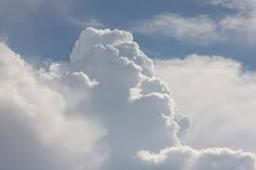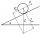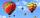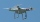# Clouds

From two points A and B on the horizontal plane was observed forehead cloud above the two points under elevation angle 73°20' and 64°40'. Points A , B are separated by 2830 m. How high is the cloud?

x =  3662.055 km

### Step-by-step explanation:Did you find an error or inaccuracy? Feel free to write us. Thank you!Tips to related online calculators
Do you have a linear equation or system of equations and looking for its solution? Or do you have a quadratic equation?
Do you want to convert length units?

#### You need to know the following knowledge to solve this word math problem:

We encourage you to watch this tutorial video on this math problem:

## Related math problems and questions:

• CloudsApproximately at what height is the cloud we see under an angle of 26°10' and see the Sun at an angle of 29°15' and the shade of the cloud is 92 meters away from us?
• Elevation anglesFrom the endpoints of the base 240 m long and inclined at an angle of 18° 15 ', the top of the mountain can be seen at elevation angles of 43° and 51°. How high is the mountain?
• AircraftThe plane flies at altitude 6500 m. At the time of first measurement was to see the elevation angle of 21° and second measurement of the elevation angle of 46°. Calculate the distance the plane flew between the two measurements.
• The bodyThe body slides down an inclined plane forming an angle α = π / 4 = 45° under the action of a horizontal plane under the effect of friction forces with acceleration a = 2.4 m/s ^ 2. At what angle β must the plane be inclined so that the body slides on it
• BearingA plane flew 50 km on a bearing 63°20' and the flew on a bearing 153°20' for 140km. Find the distance between the starting point and the ending point.
• DiagonalDetermine the dimensions of the cuboid, if diagonal long 53 dm has an angle with one edge 42° and with another edge 64°.
• Two grovesTwo groves A, B are separated by a forest, both are visible from the hunting grove C, which is connected to both by direct roads. What will be the length of the projected road from A to B, if AC = 5004 m, BC = 2600 m and angle ABC = 53° 45 ’?
• Resultant forceCalculate mathematically and graphically the resultant of three forces with a common center if: F1 = 50 kN α1 = 30° F2 = 40 kN α2 = 45° F3 = 40 kN α3 = 25°
• TrianglesFind out whether given sizes of the angles can be interior angles of a triangle: a) 23°10',84°30',72°20' b) 90°,41°33',48°37' c) 14°51',90°,75°49' d) 58°58',59°59',60°3'
• KitesBoys run kite on a cable of 68 meters long. What is the kite altitude, if the angle from the horizontal plane is 72°?
• Angles of elevationFrom points A and B on level ground, the angles of elevation of the top of a building are 25° and 37° respectively. If |AB| = 57m, calculate, to the nearest meter, the distances of the top of the building from A and B if they are both on the same side of
• Hot air balloonThe center of the balloon is at an altitude of 600 m above the ground (AGL). From habitat on earth is the center of the balloon to see in elevation angle 38°20' and the balloon is seen from the perspective of angle 1°16'. Calculate the diameter of the bal
• Right triangle trigonometricsCalculate the size of the remaining sides and angles of a right triangle ABC if it is given: b = 10 cm; c = 20 cm; angle alpha = 60° and the angle beta = 30° (use the Pythagorean theorem and functions sine, cosine, tangent, cotangent)
• A droneA flying drone aimed the area for an architect. He took off perpendicularly from point C to point D. He was at the height of 300 m above ABC's plane. The drone from point D pointed at a BDC angle of 43°. Calculate the distance between points C and B in me
• MastMast has 13 m long shadow on a slope rising from the mast foot in the direction of the shadow angle at angle 13.3°. Determine the height of the mast, if the sun above the horizon is at angle 45°12'.
• ReflectorCircular reflector throws light cone with a vertex angle 49° and is on 33 m height tower. The axis of the light beam has with the axis of the tower angle 30°. What is the maximum length of the illuminated horizontal plane?
• The rescue helicopterThe rescue helicopter is above the landing site at a height of 180m. The site of the rescue operation can be seen from here at a depth angle of 52° 40 '. How far will the helicopter land from the rescue site?